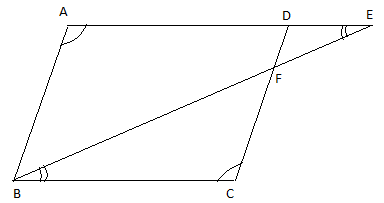QUESTION

# State true or false.E is a point on the side AD produced of a parallelogram ABCD and BE intersects CD at F. $\Delta ABE$ is similar to $\Delta CFB$.A. always trueB. may be trueC. information insufficientD. always false

Hint: Draw the figure as per said. The opposite angles of parallelogram are equal and the opposite sides are equal. Thus prove that $\Delta ABE$ is similar to $\Delta CFB$ by AA similarity criterion.

Consider the parallelogram ABCD that we have drawn. Point E is on side AD which we have produced and BE intersects CD at F. We need to find if $\Delta ABE$ is similar to $\Delta CFB$ or not.By the properties of parallelogram, opposite angles are equal.
Thus from the figure we can say that $\angle A$ is equal to the opposite angle $\angle C$.
$\therefore \angle A=\angle C.......(1)$
Also from the parallelogram ABCD, we know that opposite sides of a parallelogram are parallel, i.e. AD is parallel to BC.
$\therefore AD||BC$.
Since AE is the extended AD. AE is also parallel to BC and BE is the transversal.
$\therefore AE||BC$.
$\therefore \angle AEB=\angle CBF.......(2)$, i.e. they are alternate angles.
Now let us consider both $\Delta ABE$ and $\Delta CFB$.
From equate (1), we know, $\angle A=\angle C$.
From equate (2), we know, $\angle AEB=\angle CBF$.
Thus by AA similarity postulate we can say that $\Delta ABE$ is equal to $\Delta CFB$. Here the two angles in one triangle are congruent to two angles in another triangle, which is enough to show that both the triangles are similar.
$\therefore$By AA similarity criterion, $\Delta ABE\cong \Delta CFB$.
Hence we have proved that the given triangles are similar, i.e. the statement is true.
$\therefore$The statement is always true.
So, option A is the correct answer.

Note: The AA similarity postulate is a shortcut for showing that 2 triangles are similar. Now if you know that the two angles of the triangles are congruent, then the third angle of the triangle will also be congruent. So remember to use AA criterion in a question like this because proving the sides of a triangle is difficult in questions like this.